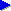Search this siteJava for beginners (topic index)Variablesfor (loops)ifelselogical operatorsarraysobjects# Java programming tutorial: variables

In pretty much any programming language, a very important idea to understand right from the are variables. A variable is essentially a value that has a name attached to it. The value of a variable can generallly be changed during the program, and the current value of the variable at any point is referred to via the variable's name.

So what are variables used for? Well, pretty much anything and everything. But as an example, let's imagine we're writing a poker game. During the game, we need to keep track of various things such as the amount of money or chips that the players have left, the number of cards still in the deck, the amount of money that has been bet so far etc. We'll also need to keep a record or count of various things temporarily. For example, in our routine to pick five random cards for a player's hand, we need a variable that counts up to five.

### Declaring a variable

The first stage in using a variable is declaring it. This basically means saying to Java "I want to create a new variable called x". For example, we might create a variable to keep track of the number of cards in the deck by declaring a variable as follows:

```int noCardsInDeck = 52;
```

If you imagine the computer memory as a big set of pigeon holes, this line effectively asks Java to pick a free pigeon hole and label the word "noCardsInDeck" to it, and then put a note inside with the number 52 written on it. At any future point in time, we can read the variable— have a look at the value of the note currently in that pigeon hole— or we can write to it— replace the note with a new note, with some different value on it.

Notice from this example a couple of features of variable declaration:

• The word int defines the type of variable. There are various available types, but a very common one is int. This stands for integer, which is the mathematical term for "whole number"— in other words, this variable will be allowed to store whole numbers (including negative numbers and zero). You may be wondering: why bother having this limitation? The answer is essentialy that by knowing that a given variable will store only whole numbers, the variable can be operated on more efficiently, and Java also then knows that it will need a certain amount of space in memory. (It turns out our pigeon holes are only so big— in some cases, we might need more than one pigeon hole for a variable!)
• We give our variable a slightly condensed name— in particular we removed spaces and abbreviated the word number to no. We'll look at this issue in more detail in the next section.

In general, a variable name should be something clear and representative, and follow certain conventions. On a separate page, we discuss Java variable name conventions in more detail; but for now, it will suffice to bear in mind this example.

### Using the variable

So now we've told Java that we want to keep a variable called noCardsInDeck, what can we do with it? Well, any time we want to read its current value, we generally just refer to it by name. For example, here is a line of code that will print out a message with the current number stored in this variable:

```System.out.println("Number of cards left: " + noCardsInDeck);
```

For now, we won't worry about what exactly System.out.println means, but compare it to the line of code that you wrote in the getting started with Java guide. Notice also that lines of Java code generally end in a semicolon (;).

If we want to set a new value for this variable, we can make a line of code that consists of the variable name, then an equals sign (=), then the new value:

```noCardsInDeck = 48;
```

Note that this time, we don't write int, because we're not declaring the variable (in other words, we're not "mentioning it for the first time").

If we want to give the variable a new value based on its current value, then we can include a calculation, such as the following, which will subtract one from the number of available cards:

```noCardsInDeck = noCardsInDeck - 1;
```

For subtracting or adding one, there is also a kind of shorthand that we can write. The following will decrement the variable, i.e. subtract one from it:

```noCardsInDeck--;
```

while the following will increment or add one to it:

```noCardsInDeck++;
```

If we want to subtract five, then either of the following lines will work (again, the second version is essentially a shorthand for the first):

```noCardsInDeck = noCardsInDeck - 5;
noCardsInDeck -= 5;
```

And assuming you have two variables defined, say, as follows:

```int noSuits = 4;
int noCardsPerSuit = 13;
```

then you can write:

```noCardsInDeck = noSuits * noCardsPerSuit;
```

Here, the multiplication symbol is the star that you get by pressing SHIFT and 8 on many US/American keyboard layouts, or that you'll find on your numeric keypad if you have one. You can't use a lower case x, for example.

## Next: control flow

On the next pages, we look at some ways in which the flow of a program is controlled: the for loop, which effectively allows us to repeat a section of code while a given variable moves over a particular range, and the if statement which lets us test a condition or make "decisions" in our program.